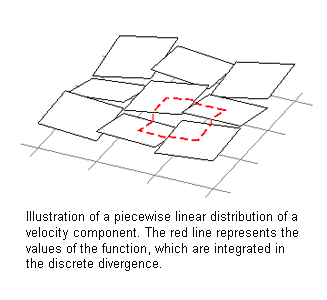Springe direkt zu Inhalt

# Numerical methods

In developing numerical techniques for computational fluid dynamics, we favor finite volume schemes in strict conservation form, [28,29], including discontinuous Galerkin schemes, , for the following reason: one ubiquitous issue with flow simulations in meteorological and enginee­ring applications is "under-resolution" of small-scale flow features. Here "under-resolution" denotes the fact that the multitude of length and time scales present in a realistic flow can generally not be represented entirely on a computational grid given today's (and tomorrow's) computational capacities. Moreover, besides the fact that it is impossible to compute all the flow details in typical applications, we are also not interested in them in general. For illustration, just consider the relevance of the flow of air around a single leaf of a tree in the context of tomorrow's weather forecast.

Now, continuum mechanics is based on the conservation principles for mass, momentum, and total energy on essentially arbitrary control volumes. These principles hold no matter how large or small the chosen control volumes are relative to the size of any flow features. Therefore, the finite-volume approach to computational fluid dynamics, which adopts the conservation laws as its fundamental construction principle, allows for underresolution by design and without loosing its justification when a flow field's representation on a given grid is non-smooth and far from being converged in the classical sense of numerical mathematics. That is, finite volume schemes provide a very solid basis for computations in the case of under-resolution, and our current efforts contribute to the development such schemes for atmospheric and engineering flow simulations.

These developments include, in particular,

- "well-balanced schemes" which correctly reproduce dominant balances of pressure and gravitational forces, [31,32],

- computational schemes for flows at small Mach and Froude numbers, [33,34,35,36]

## Computational Methods for Weakly Compressible FlowsWeakly compres­sible flows typically arise in geophysical applications, namely in atmospheric and oceanic flows, but also they are of interest for combustion problems. Mathematically, these problems are described by the Euler and Navier-Stokes equations for the conservation of mass, momentum and energy. They are characterized (in their non-dimensional form) by small Mach-, Froude-, Rossby- and Reynolds numbers. Specifically, the Mach number, which represents the ratio between the typical flow velocity of the problem and the speed of sound, describes the influence of compressibility in a given flow problem. Under certain conditions the shallow water equations are a good approximation describing the temporal evolution of flows with a small vertical extend compared to their horizontal dimension. In each horizontal point, the equations determine the temporal change of the flow depth and as well as the flow velo­city averaged over the depth of the fluid. in certain problems, velocity of surface waves becomes large to the fluid velocity itself. This is mathematically described by a small Froude number, and it is equivalent to the appearance to a small Mach number in the compressible fluid equations. A prominent example for shallow water flows with small Froude number are Tsunami waves, which propagate over whole ocean basins. However, when a Tsunami wave reaches the beach, the shallow water theory becomes very inaccurate.

- a linear momentum preserving discretization of the Coriolis term, , and

- the representation of curved flow boundaries using cartesian grid levelset-based cut-cell techniques, .

Jointly with colleagues in Germany and Swizzerland, we aim at systematic combinations of numerical discre&shy;tizations and subgrid scale process modelling within the German Research Foun&shy;dation's Priority Research Program "Multiple Scales in Fluid Mechanics and Meteorology (MetStroem)", see http://metstroem.mi.fu-berlin.de .

 LeVeque, R.J. (2002) Finite Volume Methods for Hyperbolic Problems,Cambridge University Press, Basel

[29 ]Kroener, D. (1996) Numerical Schemes for Conservation Laws, Wiley and Teubner, pp 480

 Cockburn, B (2003) Discontinuous Galerkin methods, ZAMM · Z. Angew. Math. Mech. 83, No. 11, 731 – 754

 Botta, N., Klein, R., Langenberg S., Lützenkirchen S. (2004) Well Balanced Finite Volume Methods for Nearly Hydrostatic Flows. , J. Comp. Phys., 196, 539-565

 Audusse, E. and Bouchut, F. and Bristeau, M.O. and Klein, R. and Perthame, B. (2004) A fast and stable well-balanced scheme with hydrostatic reconstruction for shallow water flows. Journal of Scientific Computation, 25 (6). pp. 2050-2065.

 Klein, R., (1995) Semi-Implicit Extension of a Godunov-Type Scheme Based on Low Mach Number Asymptotics I: One-dimensional Flow, J. Comput. Phys., 121, 213--237

 Schneider, T. and Klein, R. (1999) Overcoming Mass Losses in Level-Set-Based Interface Tracking Schemes. In: 2nd International Symposium on Finite Volumes for Complexe Applications - Problems and Perspectives, Berlin, Germany, July 19 - 22, 1999.

 Vater, S. and Klein, R. (2009) Stability of a Cartesian grid projection method for zero Froude number shallow water flows. Numerische Mathematik, 113 (1). pp. 123-161.

 Klein, R. (2009) Asymptotics, structure, and integration of sound-proof atmospheric flow equations. Theoretical and Computational Fluid Dynamics, 23 (3). pp. 161-195. ISSN 0935-4964 (Print) 1432-2250 (Online)

 Audusse, E. and Klein, R. and Owinoh, A.Z. (2009) Conservative Discretization of Coriolis Force. Journal of Computational Physics, 228 (8). pp. 2934-2950.

 Klein, R. and Bates, K.R. and Nikiforakis, N. (2009) Well Balanced Compressible Cut-Cell Simulation of Atmospheric Flow. Philosophical Transaction of the Royal Society . (In Press)

 DFG- Schwerpunktprogramm MetStroem

Complete publication list of the group